Courses

# Math - 2019 Past Year Paper

## 60 Questions MCQ Test Past Year Papers of IIT JAM Mathematics | Math - 2019 Past Year Paper

Description
This mock test of Math - 2019 Past Year Paper for IIT JAM helps you for every IIT JAM entrance exam. This contains 60 Multiple Choice Questions for IIT JAM Math - 2019 Past Year Paper (mcq) to study with solutions a complete question bank. The solved questions answers in this Math - 2019 Past Year Paper quiz give you a good mix of easy questions and tough questions. IIT JAM students definitely take this Math - 2019 Past Year Paper exercise for a better result in the exam. You can find other Math - 2019 Past Year Paper extra questions, long questions & short questions for IIT JAM on EduRev as well by searching above.
QUESTION: 1

Solution:
QUESTION: 2

Solution:
QUESTION: 3

### Let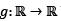be a twice differentiable function. Define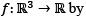f(x,y,z) = g(x2 + y2 - 2z2).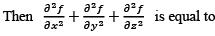Solution:
QUESTION: 4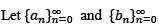be sequences of positive real numbers such that nan < bn < n2an for
all n > 2. If the radius of convergence of the power series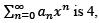then the power series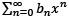Solution:

QUESTION: 5

Let S be the set of all limit points of the set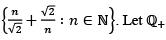be the set of all positive
rational numbers. Then

Solution:
QUESTION: 6

If xhyk is an integrating factor of the differential equation y(1 + xy) dx + x(1 — xy) dy = 0, then the ordered pair (h, k) is equal to

Solution:
QUESTION: 7

If y(x) = λe2x + eβx, β ≠ 2, is a solution of the differential equation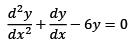satisfying dy/dx (0) = 5, then y(0) is equal to

Solution:
QUESTION: 8

The equation of the tangent plane to the surface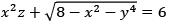at the point (2, 0, 1) is

Solution:
QUESTION: 9

The value of the integral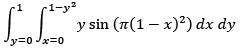is

Solution:
QUESTION: 10

The area of the surface generated by rotating the curve x = y3, 0 ≤ y ≤ 1, about the y-axis, is

Solution:
QUESTION: 11

Let H and K be subgroups of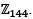If the order of H is 24 and the order of K is 36, then the order of the subgroup H ∩ K is

Solution:
QUESTION: 12

Let P be a 4 × 4 matrix with entries from the set of rational numbers. If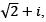with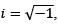is a root of the characteristic polynomial of P and I is the 4 × 4 identity matrix, then

Solution:
QUESTION: 13

The set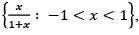as a subset of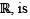Solution:
QUESTION: 14

The set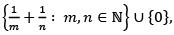as a subset of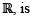Solution:
QUESTION: 15

For −1 < x < 1, the sum of the power series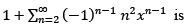Solution:
QUESTION: 16

Let f(x) = (ln x)2 , x > 0. Then

Solution:
QUESTION: 17

Let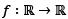be a differentiable function such that f'(x) > f(x) for all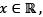and f(0) = 1. Then f( 1) lies in the interval

Solution:
QUESTION: 18

For which one of the following values of k, the equation 2x3 + 3x2 − 12x − k = 0 has three distinct real roots?

Solution:
QUESTION: 19

Which one of the following series is divergent?

Solution:
QUESTION: 20

Let S be the family of orthogonal trajectories of the family of curves 2x2 + y2 = k, for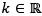and k > 0. If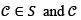passes through the point (1, 2), then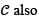passes through

Solution:
QUESTION: 21

Let x, x + ex and 1 + x + ex be solutions of a linear second order ordinary differential equation with constant coefficients. If y(x) is the solution of the same equation satisfying y(0) = 3 and y'(0) = 4, then y(1) is equal to

Solution:
QUESTION: 22

The function f(x,y) = x3 + 2xy + y3 has a saddle point at

Solution:
QUESTION: 23

The area of the part of the surface of the paraboloid x+ y2 + z = 8 lying inside the cylinder x2 + y2 = 4 is

Solution:
QUESTION: 24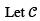be the circle (x − 1)2 + y2 = 1, oriented counter clockwise. Then the value of the line integral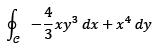is

Solution:
QUESTION: 25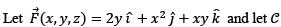be the curve of intersection of the plane x + y + z = 1 and the cylinder x2 + y2 = 1. Then the value of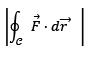is

Solution:

QUESTION: 26

The tangent line to the curve of intersection of the surface x2 + y2 − z = 0 and the plane x + y = 3 at the point (1, 1, 2) passes through

Solution:
QUESTION: 27

The set of eigenvalues of which one of the following matrices is NOT equal to the set of eigenvalues of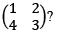Solution:
QUESTION: 28

For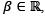, define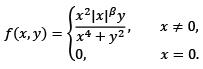Then, at (0, 0), the function f is

Solution:
QUESTION: 29

Let {an} be a sequence of positive real numbers such that a1 = 1,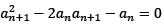for all n ≥ 1.

Then the sum of the series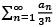lies in the interval

Solution:
QUESTION: 30

Let {an} be a sequence of positive real numbers. The series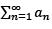converges if the series

Solution:
*Multiple options can be correct
QUESTION: 31

Let G be a noncyclic group of order 4. Consider the statements I and II:
I. There is NO injective (one-one) homomorphism from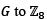II. There is NO surjective (onto) homomorphism from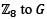Then

Solution:
*Multiple options can be correct
QUESTION: 32

Let G be a nonabelian group, y ∈ G, and let the maps f, g, h from G to itself be defined by  f(x) = yxy-1, g(x) = x-1 and h = g ° g.
Then

Solution:
*Multiple options can be correct
QUESTION: 33

Let S and T be linear transformations from a finite dimensional vector space V to itself such that S(T(v)) = 0 for all v ∈ V. Then

Solution:
*Multiple options can be correct
QUESTION: 34

Let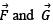be differentiable vector fields and let g be a differentiable scalar function. Then

Solution:
*Multiple options can be correct
QUESTION: 35

Consider the intervals S = (0, 2] and T = [1, 3). Let S° and T° be the sets of interior points of S and T, respectively. Then the set of interior points of S\T is equal to

Solution:
QUESTION: 36

Let {an} be the sequence given by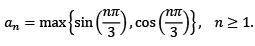Then which of the following statements is/are TRUE about the subsequences {a6n−1} and {a6n+4}?

Solution:
*Multiple options can be correct
QUESTION: 37

Let  f(x) = cos(|π - x|) + (x - n) sin |x| and g(x) = x2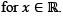If h(x) = f(g(x)), then

Solution:

*Multiple options can be correct
QUESTION: 38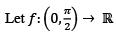be given by f(x) = (sin x)π − π sin x + π.

Then which of the following statements is/are TRUE?

Solution:
*Multiple options can be correct
QUESTION: 39

Let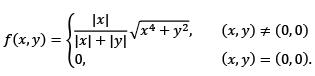Then at (0, 0),

Solution:
*Multiple options can be correct
QUESTION: 40

Let {an} be the sequence of real numbers such that a1 = 1 and an+1 = an + a2n for all n > 1.

Then

Solution:
*Answer can only contain numeric values
QUESTION: 41

Let x be the 100-cycle (1 2 3 ⋯ 100) and let y be the transposition (49 50) in the permutation group S100. Then the order of xy is ______

Solution:
*Answer can only contain numeric values
QUESTION: 42

Let W1 and W2 be subspaces of the real vector space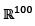defined by
W1 = {(x1,x2, ...,x100) : xi = 0 if i is divisible by 4},
W2 = { (x1;x2, ....x100) : xi = 0 if i is divisible by 5}.
Then the dimension of W1 ∩ W2 is ____

Solution:
*Answer can only contain numeric values
QUESTION: 43

Consider the following system of three linear equations in four unknowns x1, x2, x3 and x4
x1 + x2 + x3 + x4 = 4,
x1 + 2x2 + 3x3 + 4x4 = 5,
x1 + 3x2 + 5x3 + kx4 = 5.
If the system has no solutions, then k = _____

Solution:
*Answer can only contain numeric values
QUESTION: 44

Let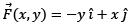and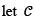be the ellipse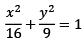oriented counter clockwise. Then the value of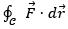(round off to 2 decimal places) is_______________

Solution:
*Answer can only contain numeric values
QUESTION: 45

The coefficient of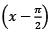in the Taylor series expansion of the function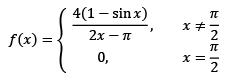about x = π/2, is ____________

Solution:
*Answer can only contain numeric values
QUESTION: 46

Let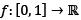be given by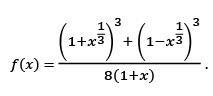Then

max {f(x): x ∈ [0,1]} - min [f(x):x ∈ [0,1]}
is ___________

Solution:
*Answer can only contain numeric values
QUESTION: 47

If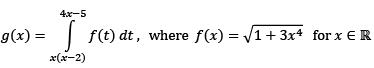then g′(1) = _______

Solution:
*Answer can only contain numeric values
QUESTION: 48

Let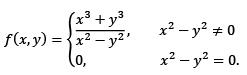Then the directional derivative of f at (0, 0) in the direction of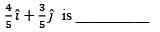Solution:
*Answer can only contain numeric values
QUESTION: 49

The value of the integral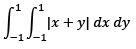(round off to 2 decimal places) is ___________

Solution:
*Answer can only contain numeric values
QUESTION: 50

The volume of the solid bounded by the surfaces x = 1 - y2 and x = y2 - 1, and the planes z = 0 and z = 2 (round off to 2 decimal places) is

Solution:
*Answer can only contain numeric values
QUESTION: 51

The volume of the solid of revolution of the loop of the curve y2 = x4 (x + 2) about the x-axis (round off to 2 decimal places) is ___________

Solution:
*Answer can only contain numeric values
QUESTION: 52

The greatest lower bound of the set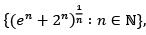(round off to 2 decimal places) is ______________

Solution:
*Answer can only contain numeric values
QUESTION: 53

Let G =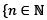: n < 55, gcd(n, 55) = 1} be the group under multiplication modulo 55. Let x ∈ G be such that x2 = 26 and x > 30. Then x is equal to _______

Solution:
*Answer can only contain numeric values
QUESTION: 54

The number of critical points of the function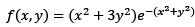is  ___________

Solution:
*Answer can only contain numeric values
QUESTION: 55

The number of elements in the set {x ∈ S3: x4 = e), where e is the identity element of the permutation group S3, is

Solution:
*Answer can only contain numeric values
QUESTION: 56

If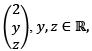is an eigenvector corresponding to a real eigenvalue of the matrix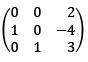then z − y is equal to__________

Solution:
*Answer can only contain numeric values
QUESTION: 57

Let M and N be any two 4 × 4 matrices with integer entries satisfying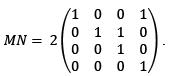Then the maximum value of det(M) + det(N) is ___________

Solution:
*Answer can only contain numeric values
QUESTION: 58

Let M be a 3 × 3 matrix with real entries such that M2 = M + 2I, where I denotes the 3 × 3 identity matrix. If α, β and γ are eigenvalues of M such that αβγ = -4, then α+β+γ is equal to ______

Solution:
*Answer can only contain numeric values
QUESTION: 59

Let y(x) = xv(x) be a solution of the differential equation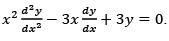If v(0) = 0 and v(1) = 1, then v(-2) is equal to ______

Solution:
*Answer can only contain numeric values
QUESTION: 60

If y(x) is the solution of the initial value problem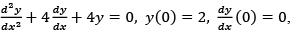then y(ln 2) is (round off to 2 decimal places) equal to ____________

Solution: